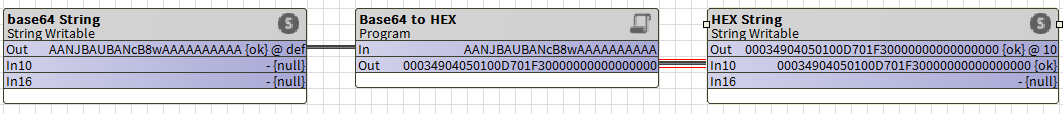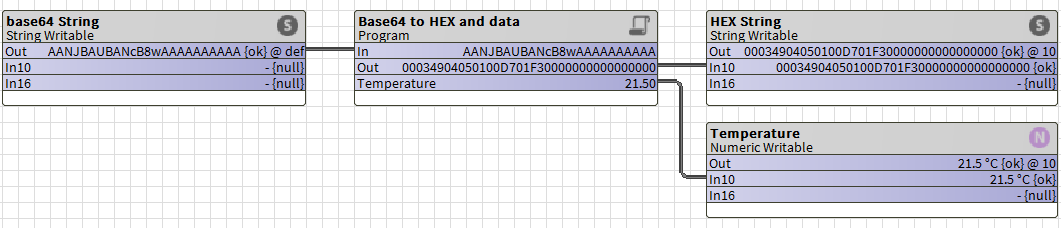1. Innon Knowledge Base
2. Tridium Niagara
3. Java, Program object quick bits

# I get data in BASE64... How do I convert it to HEX (and read data)?

## We are going to summarize the conversion between base64 and HEX, and top it up by extracting data from a bytes array

As you might have seen in other articles (like THIS ONE where we convert an HEX string to a numeric value), with the use of MQTT, REST APIs and other IoT oriented protocols, some devices might return data in a raw format, that being an hexadecimal string or even a base64 string, which Niagara would not be able to work with unless you run some conversions.

Please refer back to the article linked above if you are new to Program Objects and you have any doubt on how to drag a Program Object, how to create your Pin Slots and how to compile the code (start easy).

In this article we are going into base64 data territory, and we will show you some Java code lines that you can use in a program object within Niagara to convert the base64 value into an HEX string.

Also, we want to add more to this later if you feel you want to unleash your programming skills, by decoding a byte array into integer and singed integer values to be used in your station.

### Starting easy: transform the base64 string to an HEX string

We start by translating the Base64 string into a byte array. You might have seen THIS ARTICLE already. From Base64 though we have a function that creates the byte array in one line.

Once we have the byte array, we get a bit creative. There isn't really an "easy way" to get the byte array into an HEX string, unfortunately. Some functions that might help simplifying things (such as "javax.xml.bind.DatatypeConverter.printHexBinary") would only work on a supervisor, as it implements the full Java SE library, while a Jace supports Java Compact 3 and the set of instructions available is slightly more limited.

There are some classes we could import, but I found some code that doesn't even need that.

We create a "final" function, which is called once and creates a constant with the characters from 0 to F (HEX).

Then, I check the length of the bytes array, and multiply that by 2 to define how many characters my HEX string needs. Remember, the byte array looks like this        [D7]  [F3]         and the string will look like this "00034904050100D701F30000000000000000".

Then I start processing each character and create my char array. I cannot work directly with a string, Java doesn't allow to use "string arrays". So I convert at the end the char array into a string (Lora16), which gets eventually sent to the output.

Enough talking, let's have a look at the code, which contains all of the above, and is extracting just the temperature. The code comments (line starting with "// ") will give an indication on what that part of the code does:

`public void onStart() throws Exception{  // start up code here}public void onExecute(){// Read the base64 string input and convert it into a byte arrayString Lora = getIn();byte [] decoded = java.util.Base64.getDecoder().decode(Lora);// Translate a byte array into an HEX stringchar[] LoraChar = new char[decoded.length * 2];    for (int j = 0; j < decoded.length; j++) {        int v = decoded[j] & 0xFF;        LoraChar[j * 2] = HEX_ARRAY[v >>> 4];        LoraChar[j * 2 + 1] = HEX_ARRAY[v & 0x0F];    }String Lora16 = String.valueOf(LoraChar);// Output the HEX converted payload to an Out stringsetOut(Lora16);}public void onStop() throws Exception{  // shutdown code here}// Creates the constant we need for the string creation aboveprivate final char[] HEX_ARRAY = "0123456789ABCDEF".toCharArray();`

You need to create 2 Slot Pins for this block:

Slot "In", type "Baja - String", flags "Execute On Change" and "Summary"

Slot "Out", type "Baja - String", flags "Readonly" and "Summary"

Here it is being used in a wire sheet### Add extra complexity: extract numeric values from the code above

You might have noticed we have an article extracting data (like "temperature" etc) directly from an HEX string, available HERE. So, what if we wanted to apply the same concept to this code?

We have a bytes array (called "decoded") available already. Let's take an example using the Enless sensor and the same code of the article linked above.

I can check the Enless documentation (in this case there is an Excel sheet where I can check the meaning of each byte for each sensor). The document tells me that bytes 6 and 7 ( [D7]) are my temperature (decimal value 215, divide by 10 is 21.5 degrees Celsius), and that bytes 8 and 9 ( [F3]) are my humidity (decimal value 499, divide by 10 is 49.9%).

In Java then, I can extract those bytes and create a new "sliced" array with just the 2 bytes I need.

I then need to create a formula that puts together those 2 bytes and returns me an integer number. I had to create 2 formulas: one that just puts together the 2 bytes to return an unsigned 16 bits integer, and then a second one that checks for bit 15 and converts the number into a signed integer. Depending on the data you need to extract (i.e. temperature is signed, CO2 level would be unsigned) you can use the right one for you.

Note that I had to always filter the data with "AND FF". The reason is Java uses signed byte notation on byte arrays (-128 to 127 instead of 0 to 255), so filtering it by AND each bit with FF I use it as unsigned.

Enough talking, let's have a look at the code, which contains all of the above, and is extracting just the temperature. The code comments (line starting with "// ") will give an indication on what that part of the code does:

`public void onStart() throws Exception{  // start up code here}public void onExecute(){// Read the base64 string input and convert it into a byte arrayString Lora = getIn();byte [] decoded = java.util.Base64.getDecoder().decode(Lora);// Translate a byte array into an HEX stringchar[] LoraChar = new char[decoded.length * 2];    for (int j = 0; j < decoded.length; j++) {        int v = decoded[j] & 0xFF;        LoraChar[j * 2] = HEX_ARRAY[v >>> 4];        LoraChar[j * 2 + 1] = HEX_ARRAY[v & 0x0F];    }String Lora16 = String.valueOf(LoraChar);// Output the HEX converted payload to an Out stringsetOut(Lora16);// slice from index 6 to index 8, and then run the formula to put the 2 bytes together into a signed valuebyte[] sliced = Arrays.copyOfRange(decoded, 6, 8);double temperature = readInt16LE(sliced) / 10d;setTemperature(temperature);}public void onStop() throws Exception{  // shutdown code here}// Code to create unsigned data from 2 bytespublic long readUInt16LE(byte[] bytes) {    long value = ((bytes & 0xFF) << 8) + (bytes & 0xFF);    return value & 0xFFFF;}// Code checking bit 15 of my unsigned data and transform it into a signed valuepublic double readInt16LE(byte[] bytes) {    double ref = readUInt16LE(bytes);    return ref > 0x7fff ? ref - 0x10000 : ref; }// Creates the constant we need for the string creation aboveprivate final char[] HEX_ARRAY = "0123456789ABCDEF".toCharArray();`

You need to create 3 Slot Pins for this block:

Slot "In", type "Baja - String", flags "Execute On Change" and "Summary"

Slot "Out", type "Baja - String", flags "Readonly" and "Summary"

Slot "Temperature", type "Baja - Double", flags "Readonly" and "Summary"

Here it is being used in a wire sheet